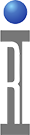Measurement of Differential S-parameters

Many devices have differential inputs or outputs. The RI Cassini tester is able to measure these devices directly by committing separate RF ports to each arm of the differential input or output. The separate measurement of each port is combined with mathematics to provide common-mode and differential-mode S-parameters for the differential ports.

The measurement of S-parameters starts with a good calibration. In this case, we are going to measure a demo device with connectors, we will use an Engineering fixture on the Cassini tester and we will perform a fixture calibration at the end of the cables used to connect the device. This includes any loss or length from the cables in the calibration, thus removing it from the measurement data.

Here is an image of our test device connected to the Cassini Engineering Fixture. Port RF3 is used on the single-ended input and ports RF6 and RF7 are used for the differential output of the test device.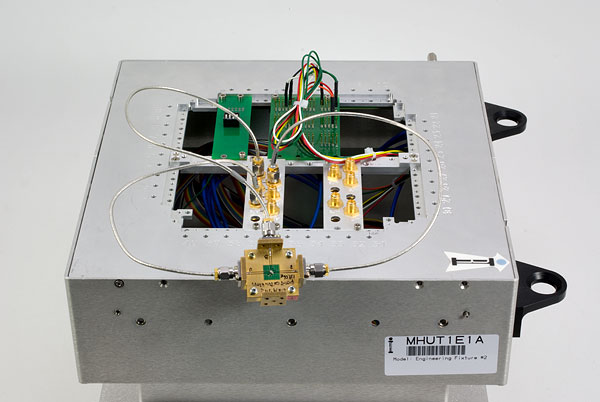The calibrations must be performed in a specific order:

First perform one-port calibrations at the end of each test cable. Each one-port cal requires the connection of the open, short, and load device. An example is shown below. This is repeated for each calibration standard and at each port.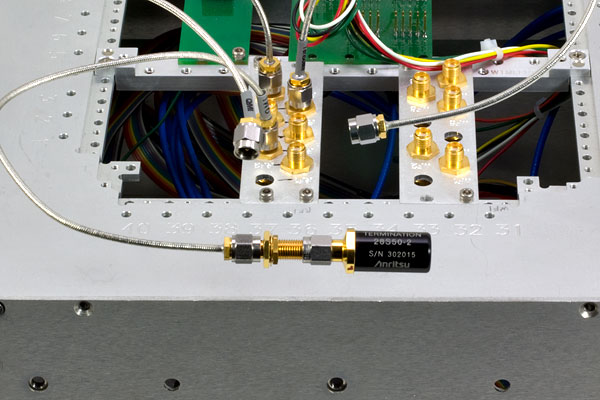Next perform two-port "thru" calibrations between each of the port pairs, e.g. RF3 to RF6, RF3 to RF7, and RF6 to RF7. This layer of the calibration modifies the one-port calibrations already performed, which is why the one-port cals must be done first. An example of a thru cal is shown below.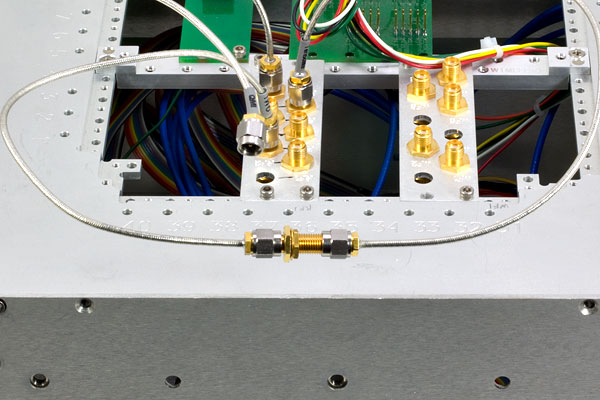After performing all fixture calibrations, remember to save the calibration before moving on.

The measurement of differential S-parameters is carried out by first characterizing the device with full 2-port measurements for each pair of ports. Shown below is one path of three for our example device -- a filter that has a single-ended input and differential output. In our device, the single ended port is connected to RF3 and the differential outputs are connected to RF6 and RF7.

For this test device, the bench data was taken with some length of cable attached. Because we have removed the electrical length of our own cables through calibration, we need to add electrical length to our measurement to match bench data. This is often called "reference plane extension" on benchtop network analyzers and is performed here by calculating a fixed delay and adding it to the measurement.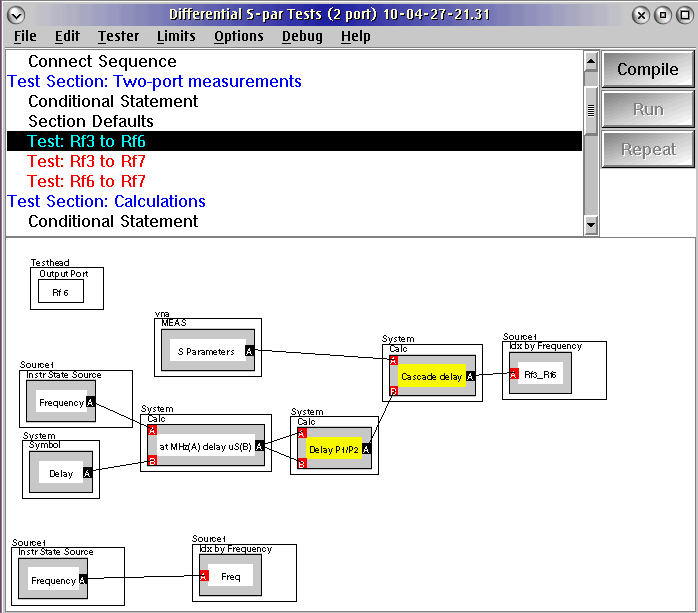After the data for all three paths is acquired (RF3 to RF6, RF3 to RF7, and RF6 to RF7), we have everything we need to calculate the 3-port equivalents of this device. Using the method outlined in the Anritsu application note "Three and Four Port S-Parameter Measurements" the calculations are done in three sections. First, the single-ended reflection is calculated for port RF3. This calculation is unmodified from the two-port measurment (as you would expect, since the input is single-ended).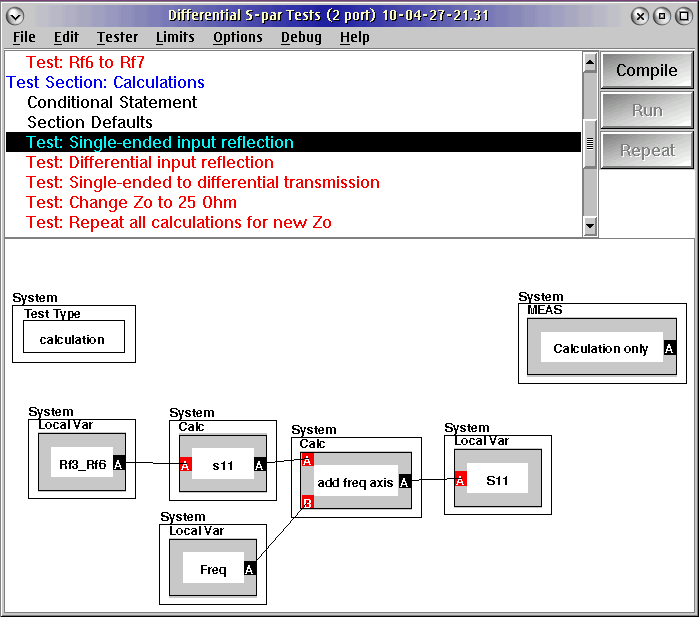Next, the reflections are calculated for the differential ports, RF6 and RF7. This calculation involves only the two-port measurement of RF6 to RF7 and results in the common-mode and differential-mode reflection for the port. The appendix of the Anritsu application note details the expression which is shown here in blocks.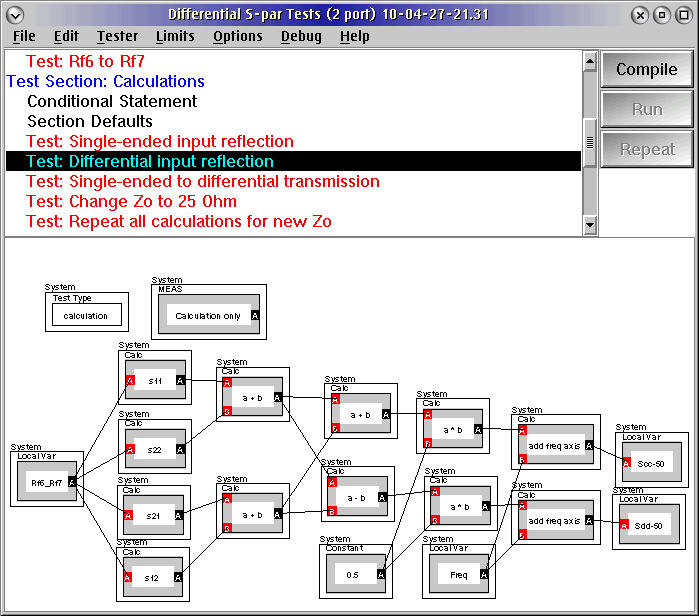Next we calculate the transfer S-parameters from the single-ended input to the differential output. This involves the measurements for RF3 to RF6 and RF3 to RF7. This results in a single-ended to common-mode result and a single-ended to differential-mode result. A tracking term is also calculated in this case to check the balance of the two paths through the device.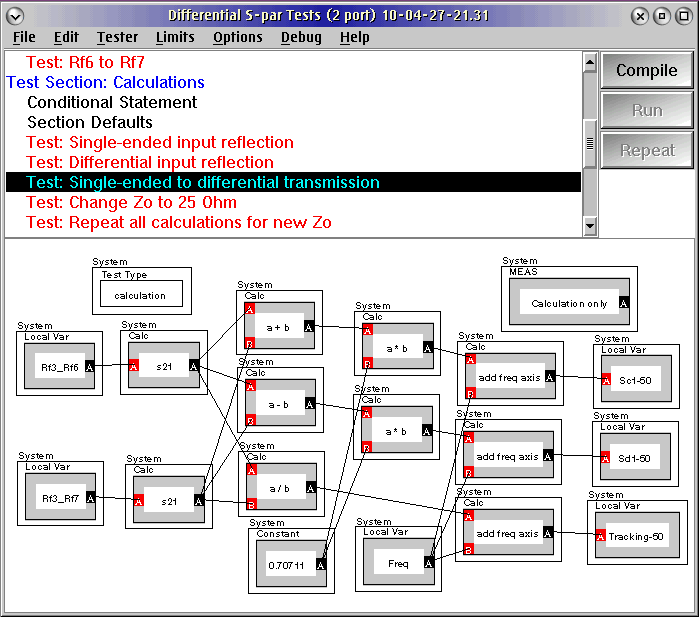This technique can be extended to devices with differential input and output by measuring more two-port paths and performing additional calculations. One last item to consider is the transformation to non-traditional characteristic impedances. In many cases the device's characteristic impedance is not the same as the tester (50 ohms). In this case it is sometimes useful to transform the measured S-parameters to another characteristic impedance. The technique is shown below.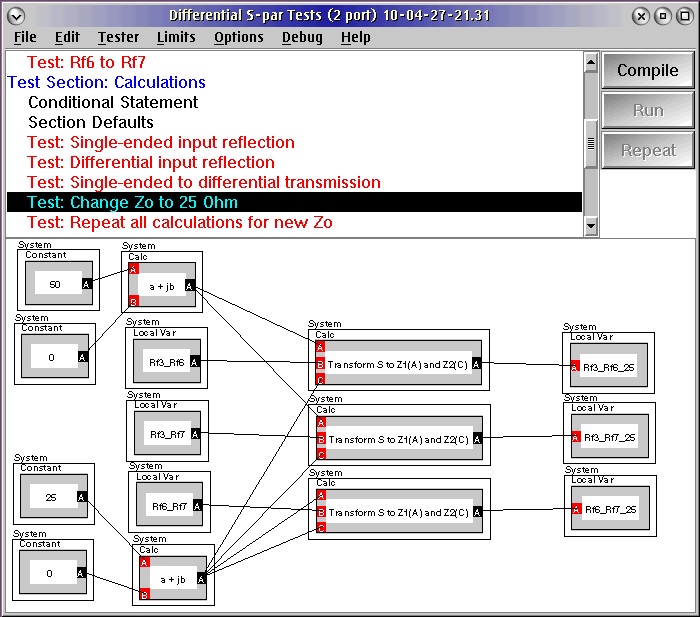After transforming the measured S-parameters to the new characteristic impedance, the same differential S-parameter calculations may be done.

https://roos.com/docs/JBAT-84X7DR
ROOS INSTRUMENTS CONFIDENTIAL AND PROPRIETARY